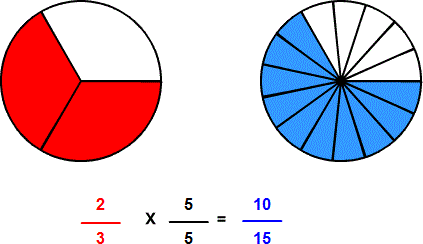# Rename higher with circle models

## Learn or teach by designing your own rename to higher terms visual examples. All examples are modeled with circles.

RENAME HIGHER WITH CIRCLE MODELS
SHOW COLOR
EXPLAIN
SHOW INPUT
LOWER TERMS TO HIGHER TERMS

# INSTRUCTIONS

Rename to Higher Terms with Circle Models uses circles to demonstrate how a fraction can be renamed to higher terms.

The following equivalent fractions were made from Rename to Higher Terms:When the program starts, you will be shown two fractions. The fractions are equivalent, but both the numerator and denominator of the fraction on the right are in higher terms.

The example shows that the fraction on the left will not change in value if the numerator and denominator are multiplied by the same number. In the above image, both the numerator and denominator are multiplied by 4.

Although we chose a multiplier,4 we are multiplying the fraction by 4/4, a form of one. One (1) is called the multiplicative identity because multiplying a number by one or some form of one does not change the value of the number.

You can input a fraction less than one and a multiplier less than 100.

On the left is a <SHOW COLOR> button. Uncheck the button to turn off the red and blue parts on the circles. This will allow the learner to demonstrate the size of the fraction.

Uncheck the <EXPLAIN> check box to turn off the answer and the explanation. You can ask your students to complete the number sentence.

With <EXPLAIN> and <SHOW INPUT> unchecked you can ask your students to write a number sentence that explains the picture.

With <EXPLAIN> and <SHOW COLOR> unchecked you can ask your students to shade the indicated fraction and write in higher terms.

Suggestions:

Turn color and explain off and demonstrate how much of the circle should be shaded. Then write the fraction in higher terms.

Keep the first fraction the same size, but increase the size of the multiplier. Observe that there are more parts, but the parts are smaller.

Emphasize that if you select 4 for a multiplier, you are not multiplying by 4, but by 44 , a form of one. One (1) is the identity for multiplication. A fraction multiplied by one(1) or a form of one results in an equivalent fraction.

WINDOWS COMPUTERS

Windows users can select any part of the screen by right clicking and selecting "Take a screenshot". Adjust to fit mage you want. This copies the selection into Windows Clipboard™. The screen can then be pasted into Windows Paint™ or your favorite imaging program. Or you can select "Download" which will put the image into your files "Download" folder.